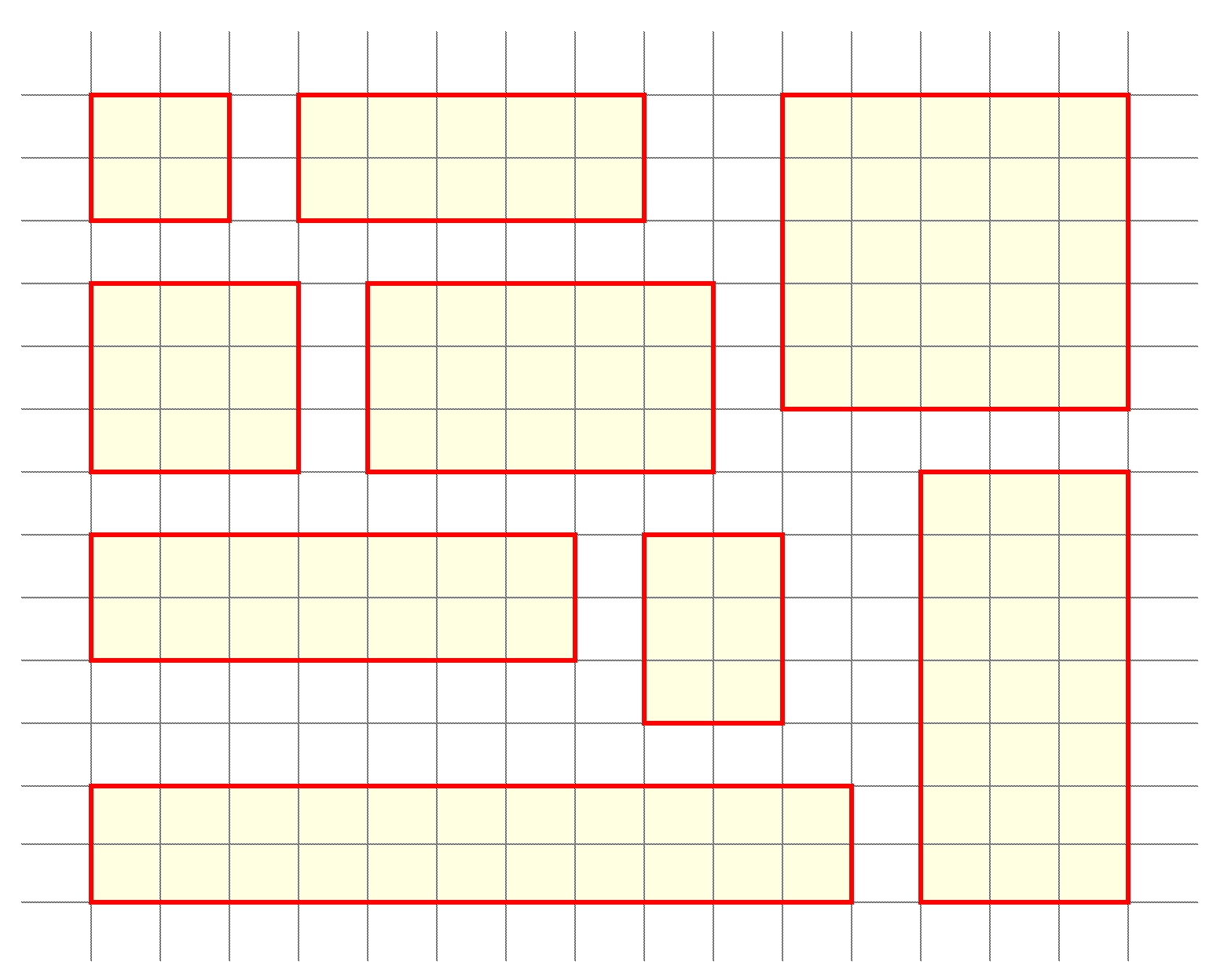# Problem 52724. Easy Sequences 20: Counting Prime-sided Rectangles

A prime-sided rectangle is a rectangle having sides represented by prime numbers. The figure below shows all the possible prime-sided rectangles whose areas are less than or equal to 25:Given an area limit 'n', count the total number of prime-sided rectangles that can be formed , with areas less than or equal to 'n'.
In the figure above, we can see that there are only 9 prime-sided recatangles having areas are less than or equal to 25. Therefore, for n = 25 the output should be 9. For n = 100, there are 34 such rectangles.
NOTE: Rotations are not important and are counted only once.

### Solution Stats

75.0% Correct | 25.0% Incorrect
Last Solution submitted on Mar 17, 2023

### Community Treasure Hunt

Find the treasures in MATLAB Central and discover how the community can help you!

Start Hunting!